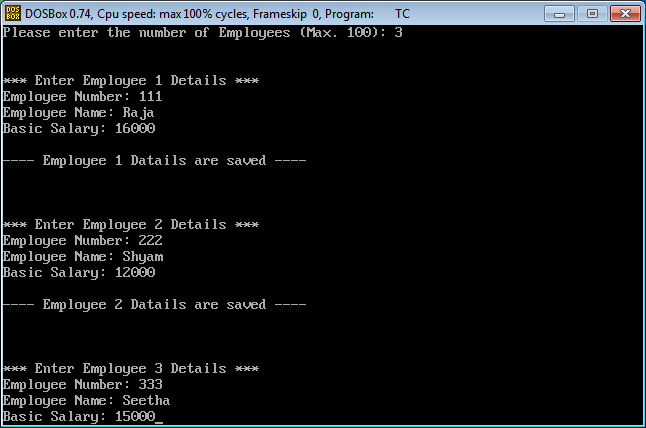The perfect place for easy learning...

×

# Aim

Write a C++ program to read data of N employee and computer net salary of each employee (DA = 52% of Basic and IT = 30% of the gross salary).

# Procedure

• Step 1 - Include the required header files (iostream.h, conio.h, and windows.h for colors).
• Step 2 - Create a class Employee with the following members.
• emp_number, emp_name, basic, da, it, gross_salary, and net_salary as data memebrs
• read_emp_details(), find_net_salary(), and display_emp_details() as member functions.
• Step 3 - Implement all the member functions with their respective code.
• Step 4 - Create a main() method.
• Step 5 - Create an array of class object with a specific size and number_of_emp as integer.
• Step 6 - Read number_of_emp.
• Step 7 - Call the read_emp_details() method through the array of class object from 0 to number_of_emp.
• Step 8 - Call the find_net_salary() method through the array of class object from 0 to number_of_emp.
• Step 9 - Call the display_emp_details() method through the array of class object from 0 to number_of_emp.
• Step 10 - returnn 0 to exit form the program execution.

# Implementation

C++ Program
``````/*
Write a C++ program to read data of N employee and computer net salary of each employee
(DA = 52% of Basic and IT = 30% of the gross salary)
*/
#include<iostream.h>
#include<conio.h>

class Employee
{
char emp_name;
int emp_number;
float basic, da, it, gross_salary, net_salary;
public:
cout<<"\n\n*** Enter Employee "<<count<<" Details ***";
cout<<"\nEmployee Number: ";
cin>>emp_number;
cout<<"Employee Name: ";
cin>>emp_name;
cout<<"Basic Salary: ";
cin>>basic;
cout<<"\n---- Employee "<<count<<" Datails are saved ----\n\n";
}
float find_net_salary(){
da = basic * 0.52;
gross_salary = basic + da;
it = gross_salary * 0.30;
net_salary = (basic + da) - it;
return net_salary;
}
void display_emp_details(int count){
cout<<"\n\n*** Employee "<<count<<" Details ***\n";
cout<<"\nEmployee Number	: "<<emp_number;
cout<<"\nEmployee Name	: "<<emp_name;
cout<<"\nNet Salary: "<<net_salary;
cout<<"\n--------------------------\n";
}
};
int main(){
Employee emp;
int number_of_emp, count;
clrscr();
cout<<"\nPlease enter the number of Employees (Max. 100): ";
cin>>number_of_emp;
for(count=0; count< number_of_emp; count++){
}
for(count=0; count < number_of_emp; count++){
emp[count].find_net_salary();
}
for(count=0; count < number_of_emp; count++){
emp[count].display_emp_details(count+1);
}
cout<<"\nPress any key to close!!!";
getch();
return 0;
}
```
```

# Result